Study Guide

# Parallelograms

## Parallelograms

Parallelograms are everywhere. They hide in plain sight, staring us straight in the face. Like towel rods and toilets, we use them every day and never give them much thought. Right now, as you read this, you are looking at a parallelogram. Yeah, that's right. Your computer screen is a parallelogram. Unless you have a particularly wonky-looking screen, that is.

A parallelogram is any quadrilateral with two sets of parallel sides. When we think of parallelograms, we usually think of something like this.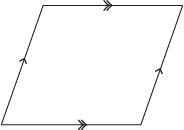Unless you did some serious damage to your computer screen, it probably doesn't look like that. For the good of your bank account, we sincerely hope it doesn't look like that. It should be rectangular. So what gives?

Well, a rectangle has two sets of parallel sides, doesn't it? So it's just as much of a parallelogram as the one above. In fact, squares, rhombi, and rectangles are all special cases of parallelograms.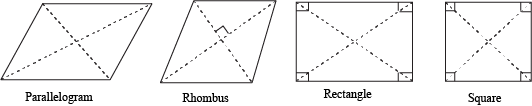Don't you worry your pretty little head about those specific parallelograms just yet. We'll cover those in more detail later on.

Since parallelograms are as common as a brain freeze at an ice cream shop, let's get down and dirty with them. Only not in the ice cream shop. That's just unsanitary.

Since a parallelogram is a specific type of quadrilateral, it comes with all the goodies that quadrilaterals have, like 360° of internal angles, opposite angles, sides, and vertices, and two honorable and generous diagonals.

Yes, we did just refer to those diagonals as "honorable and generous," and it's not because they donate to charities or volunteer at soup kitchens. It's because the diagonals of a parallelogram split it up into two congruent triangles.

Don't believe us? We can prove it. Can you?

### Sample Problem

Given that ABCD is a parallelogram, prove that ΔABC and ΔCDA are congruent.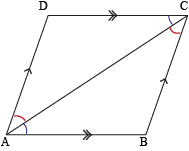Statements Reasons 1. ABCD is a parallelogram Given 2. AB || CD and BC || DA Definition of a parallelogram (1) 3. ∠BAC ≅ ∠DCA, ∠BCA ≅ ∠DAC Alternate interior angles theorem (3) 4. AC ≅ AC Reflexive property 5. ΔABC ≅ ΔCDA ASA Postulate (3, 4)

Bam! Congruent triangles. We don't want to say we told you so, so we'll opt for, "We informed you thusly."

But you know what's even better? Thanks to CPCTC (Corresponding Parts of Congruent Triangles are Congruent), we can prove three more theorems right away:

1. Both pairs of opposite sides of a parallelogram are congruent. So for ABCD, ABCD and BCDA.
2. Both pairs of opposite angles are congruent So for ABCD, ∠B ≅ ∠D and ∠A ≅ ∠C.
3. Two consecutive angles are supplementary. So ∠A and ∠B are supplementary, as are ∠C and ∠D.

With all of these theorems about parallelograms, it's like we struck mathematical gold. But wait, there's more! Order in the next ten minutes and we'll throw in another theorem, absolutely free! Just pay \$4.95 for shipping and handling. Or, if you'd rather not, you can get the theorem for free! Just handle it yourself.

### Sample Problem

Given that ABCD is a parallelogram, prove that its diagonals, AC and BD, bisect each other.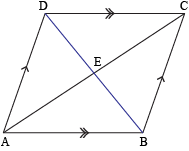Statements Reasons 1. ABCD is a parallelogram Given 2. ∠BAE ≅ ∠DCE and ∠ABE ≅ ∠CDE Alternate interior angles theorem (1) 3. AB ≅ CD Opposite sides of a parallelogram are congruent (1) 4. ΔABE ≅ ΔCDE ASA Postulate (2, 3) 5. AE ≅ CE and BE ≅ DE CPCTC (4) 6. E is the midpoint of AC and BD Definition of midpoint (5) 7. AC and BD bisect each other Definition of segment bisector (6)

The key to this proof (and probably most proofs about quadrilaterals) is a theorem about triangles. Oh, you thought they were over and done with, did you? Well, we hate to burst your bubble, bud, but we learned about those triangles for a reason. And it wasn't just to make your math teacher happy.

We've established five different theorems about parallelograms. So it's only reasonable that we ask our favorite questions about mathematics. "Why do we care?" "What does this matter?" "How is this at all relevant to my life?" "Why are these little golden nuggets of wisdom important?"

Well, we could continue collecting these nuggets and eventually give Fort Knox a run for its money. On the flip side, we'd be hoarding a bunch of useless facts when we could be using them as parallelogram primers.

Let's say you've accused a quadrilateral of being a parallelogram. Now you need to state your case. Here are some ways you can convince the jury that the quadrilateral is guilty of being a parallelogram:

1. The two pairs of opposite sides are parallel. (This is the definition of a parallelogram.)
2. Both pairs of opposite sides are congruent.
3. One pair of opposite sides is both congruent and parallel.
4. Both pairs of opposite angles are congruent.
5. The diagonals bisect each other.

Many of these statements are the converse statements of the proofs we came up with already. Before, we assumed a figure was a parallelogram, and we showed that the figure had some special properties. Now, we're saying that if the figure has these properties, it must be a parallelogram. Aside from the definition, we should show each of these statements is true. Lucky for us, we just need to apply the facts we already know.

### Sample Problem

Given: Quadrilateral ABCD has two pairs of opposite sides that are congruent.
Prove: ABCD is a parallelogram.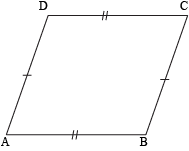Statements Reasons 1. AB ≅ CD and BC ≅ DA Given 2. Diagonal AC creates ΔABC and ΔCDA Definition of a triangle 3. AC ≅ AC Reflexive property 4. ΔABC ≅ ΔCDA SSS Postulate (1, 2, 3) 5. ∠BAC ≅ ∠DCA and ∠CAD ≅ ∠ACB CPCTC (4) 6. AB || CD and BC || DA Converse alternate interior angles theorem (5) 7. ABCD is a parallelogram Definition of a parallelogram (6)

We can prove the rest of the theorems in similar ways, but we aren't going to right now. We'll leave that fun for later. Let's throw some numbers into the mix.

### Sample Problem

What are the lengths of FJ and JH in parallelogram FGHJ?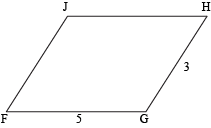Since opposite sides of a parallelogram are congruent, we know that FJGH and that GH = 3. That means FJ = 3, too. Same goes for JH, only with FG, which has a length of 5. So JH = 5 as well.